CBSE Class 12 Sample Paper for 2021 Boards

Class 12
Solutions of Sample Papers and Past Year Papers - for Class 12 Boards

## Find the foot of the perpendicular drawn from the point (-1, 3, -6) to the plane 2𝑥 + 𝑦 − 2𝑧 + 5 = 0. Also find the equation and length of the perpendicular.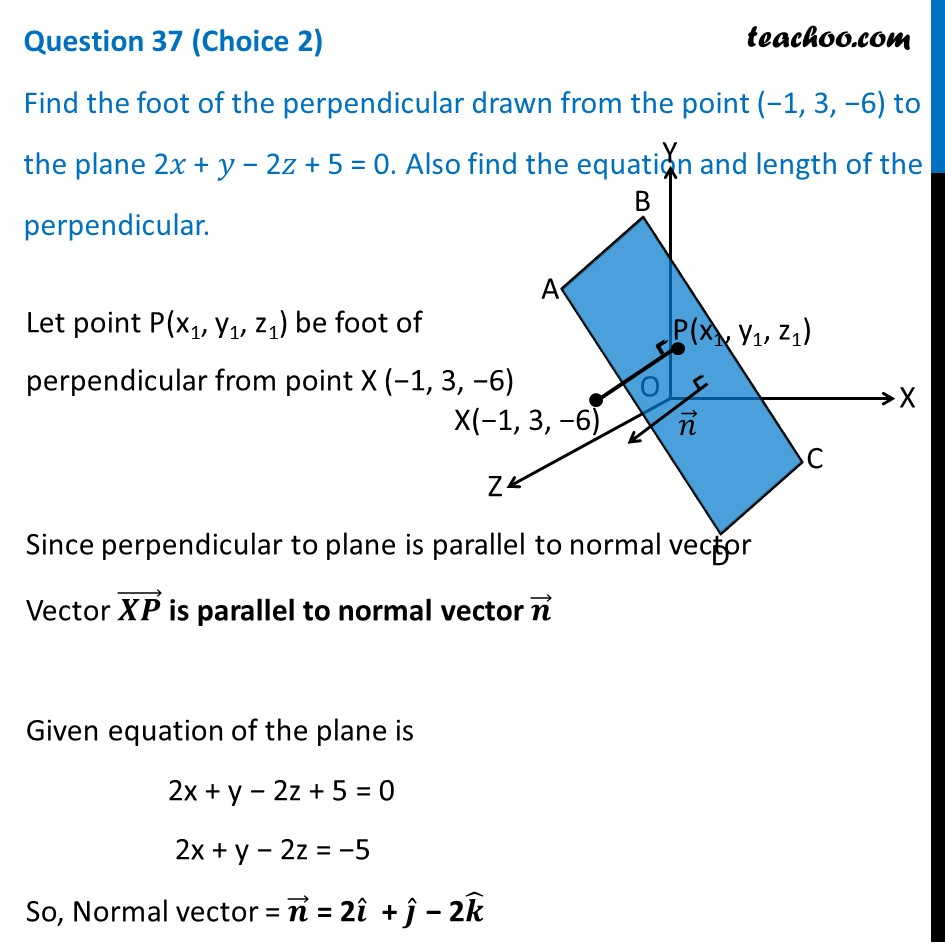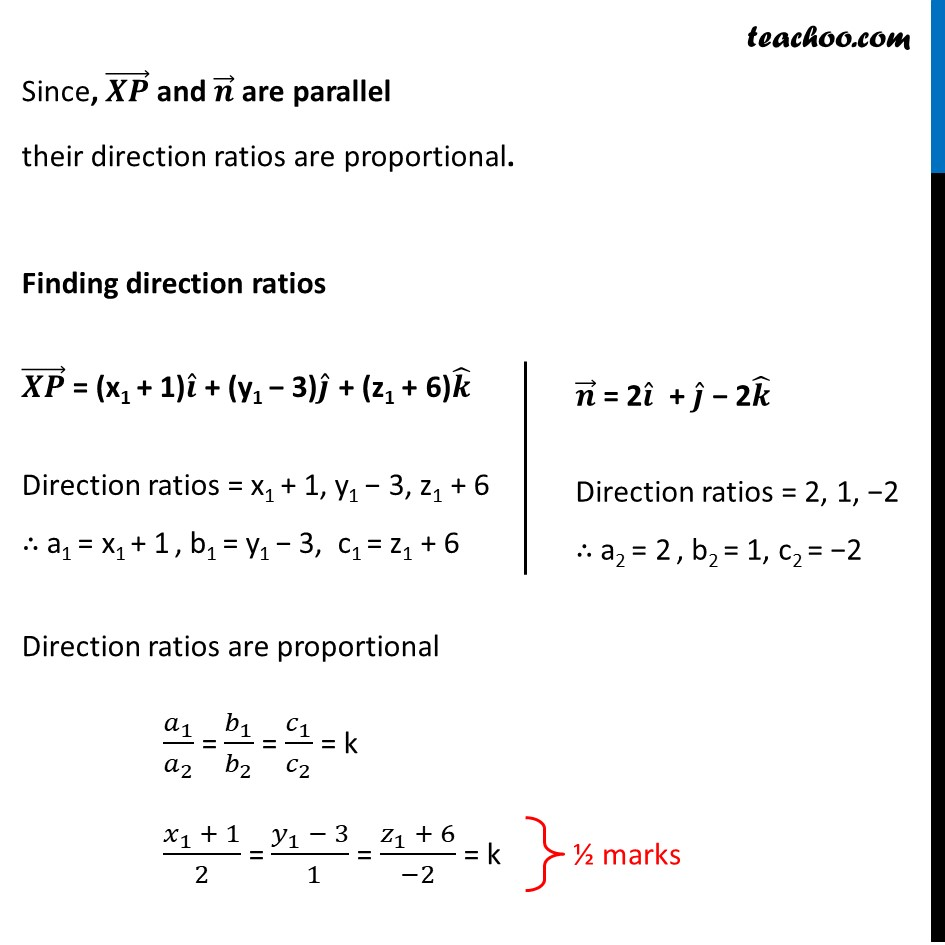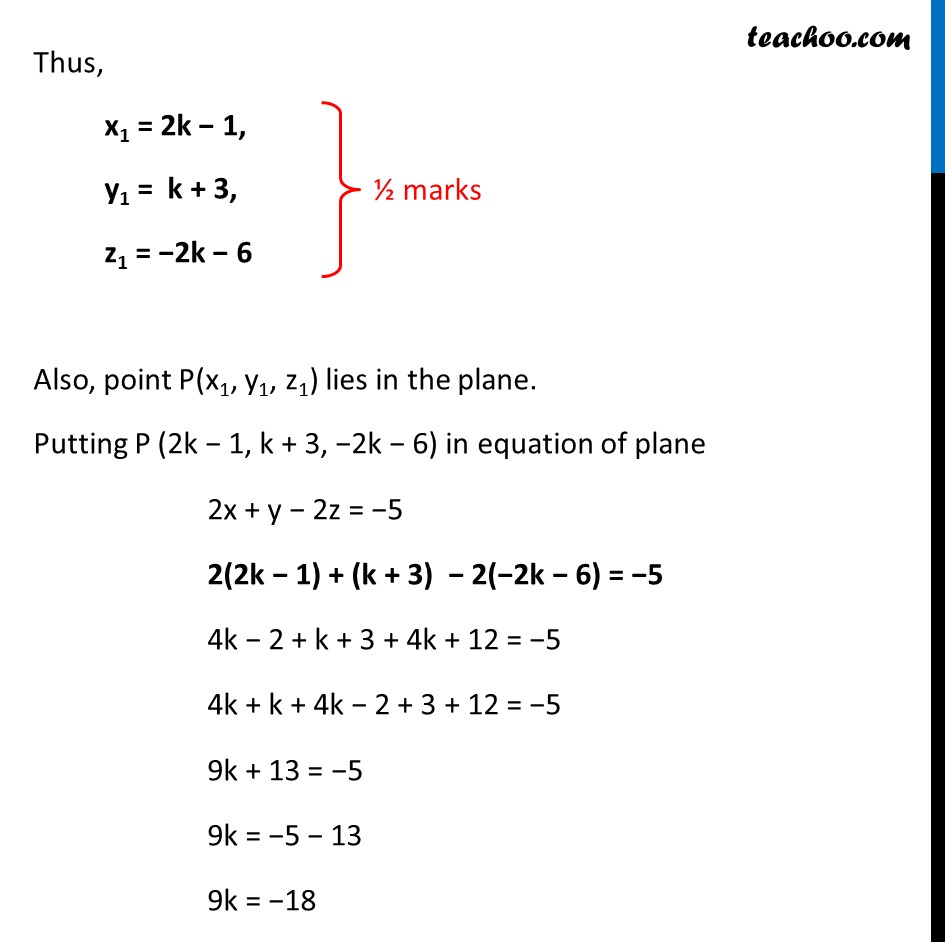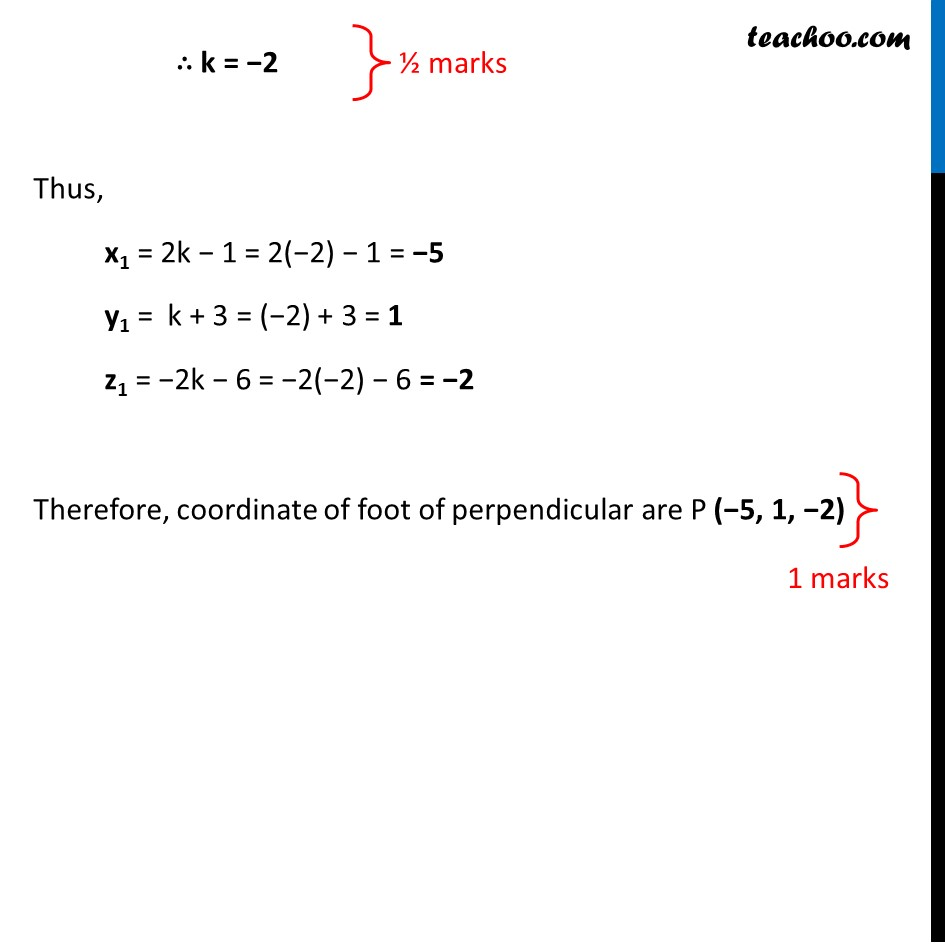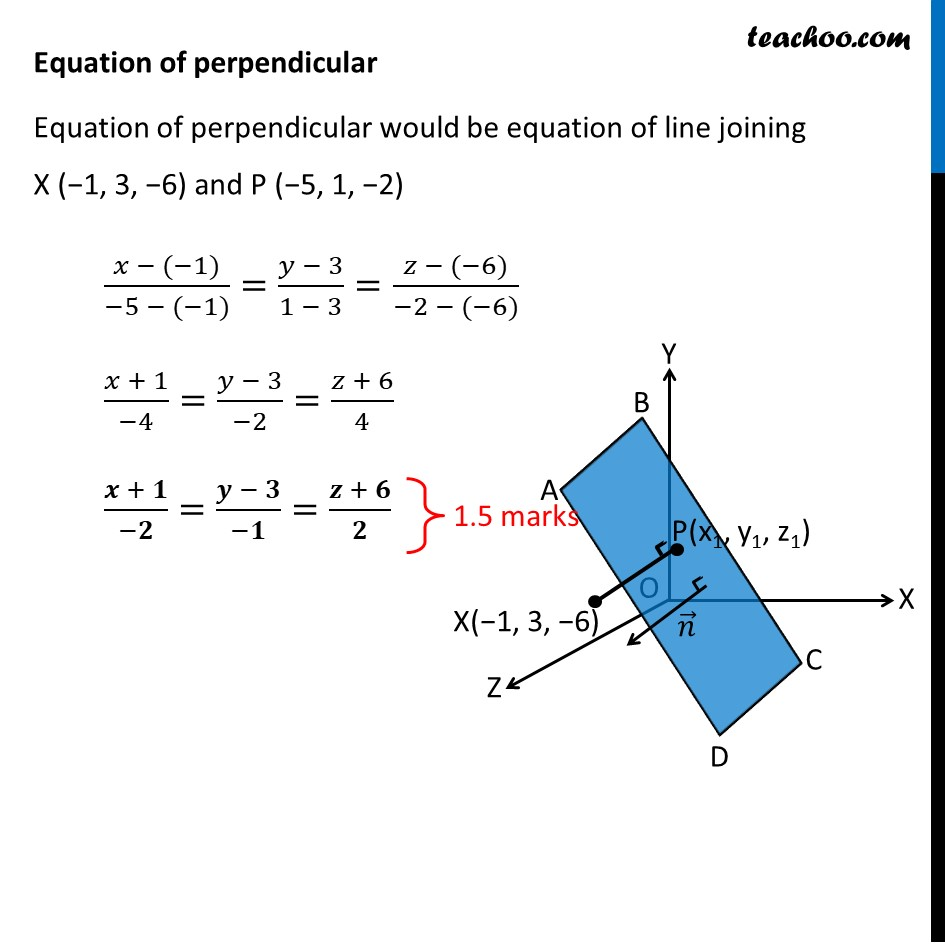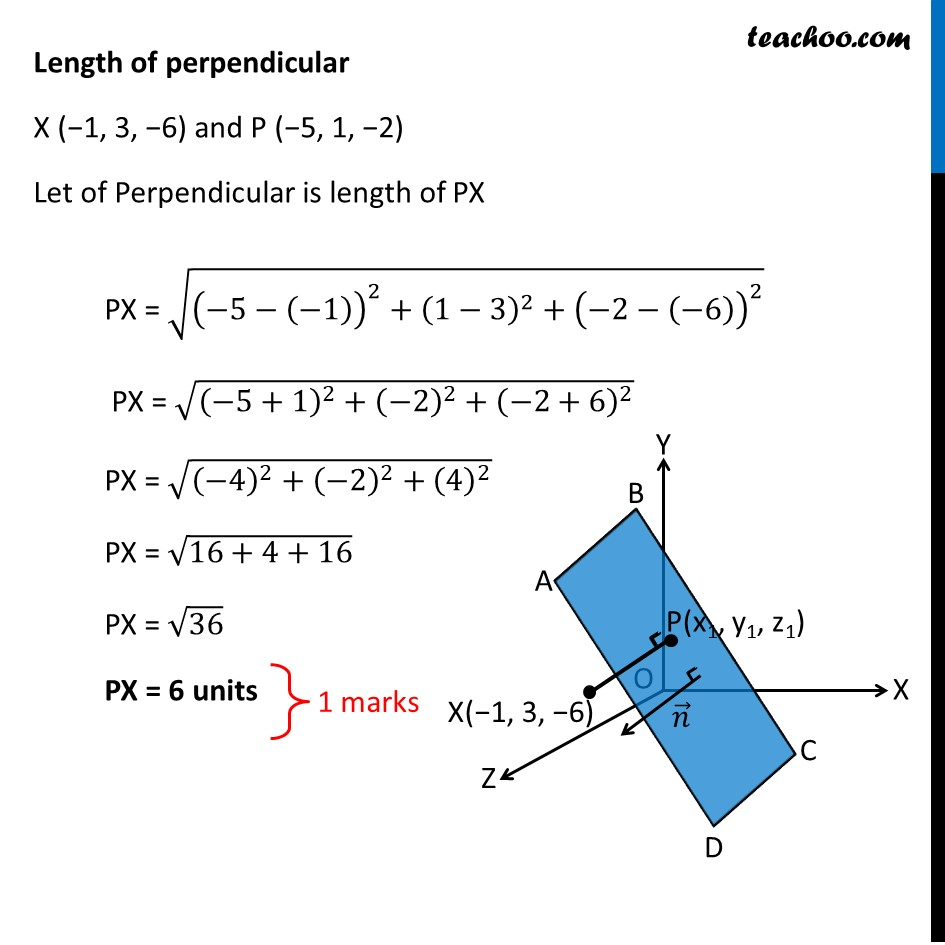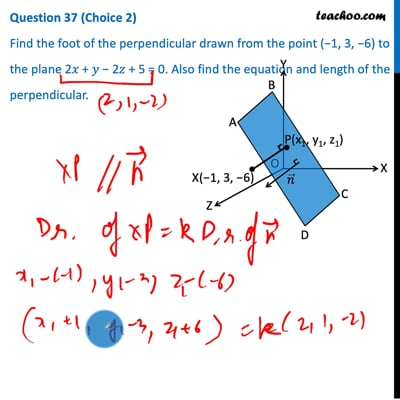This video is only available for Teachoo black users

Note : This is similar to Example 16 of NCERT – Chapter 11 Class 12 Three Dimensional Geometry

Introducing your new favourite teacher - Teachoo Black, at only ₹83 per month

### Transcript

Question 37 (Choice 2) Find the foot of the perpendicular drawn from the point (−1, 3, −6) to the plane 2𝑥 + 𝑦 − 2𝑧 + 5 = 0. Also find the equation and length of the perpendicular. Let point P(x1, y1, z1) be foot of perpendicular from point X (−1, 3, −6) Since perpendicular to plane is parallel to normal vector Vector (𝑿𝑷) ⃗ is parallel to normal vector 𝒏 ⃗ Given equation of the plane is 2x + y − 2z + 5 = 0 2x + y − 2z = −5 So, Normal vector = 𝒏 ⃗ = 2𝒊 ̂ + 𝒋 ̂ − 2𝒌 ̂ Since, (𝑿𝑷) ⃗ and 𝒏 ⃗ are parallel their direction ratios are proportional. Finding direction ratios (𝑿𝑷) ⃗ = (x1 + 1)𝒊 ̂ + (y1 − 3)𝒋 ̂ + (z1 + 6)𝒌 ̂ Direction ratios = x1 + 1, y1 − 3, z1 + 6 ∴ a1 = x1 + 1 , b1 = y1 − 3, c1 = z1 + 6 𝒏 ⃗ = 2𝒊 ̂ + 𝒋 ̂ − 2𝒌 ̂ Direction ratios = 2, 1, −2 ∴ a2 = 2 , b2 = 1, c2 = −2 Direction ratios are proportional 𝑎_1/𝑎_2 = 𝑏_1/𝑏_2 = 𝑐_1/𝑐_2 = k (𝑥_1 + 1)/2 = (𝑦_1 − 3)/( 1) = (𝑧_1 + 6)/(−2) = k Thus, x1 = 2k − 1, y1 = k + 3, z1 = −2k − 6 Also, point P(x1, y1, z1) lies in the plane. Putting P (2k − 1, k + 3, −2k − 6) in equation of plane 2x + y − 2z = −5 2(2k − 1) + (k + 3) − 2(−2k − 6) = −5 4k − 2 + k + 3 + 4k + 12 = −5 4k + k + 4k − 2 + 3 + 12 = −5 9k + 13 = −5 9k = −5 − 13 9k = −18 ∴ k = −2 Thus, x1 = 2k − 1 = 2(−2) − 1 = −5 y1 = k + 3 = (−2) + 3 = 1 z1 = −2k − 6 = −2(−2) − 6 = −2 Therefore, coordinate of foot of perpendicular are P (−5, 1, −2) Equation of perpendicular Equation of perpendicular would be equation of line joining X (−1, 3, −6) and P (−5, 1, −2) (𝑥 − (−1))/(−5 − (−1))=(𝑦 − 3)/(1 − 3)=(𝑧 − (−6))/(−2 − (−6)) (𝑥 + 1)/(−4)=(𝑦 − 3)/(−2)=(𝑧 + 6)/4 (𝒙 + 𝟏)/(−𝟐)=(𝒚 − 𝟑)/(−𝟏)=(𝒛 + 𝟔)/𝟐 Length of perpendicular X (−1, 3, −6) and P (−5, 1, −2) Let of Perpendicular is length of PX PX = √((−5−(−1))^2+(1−3)^2+(−2−(−6))^2 ) PX = √((−5+1)^2+(−2)^2+(−2+6)^2 ) PX = √((−4)^2+(−2)^2+(4)^2 ) PX = √(16+4+16) PX = √36 PX = 6 units